Anúncio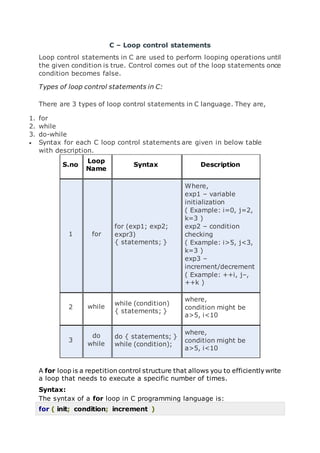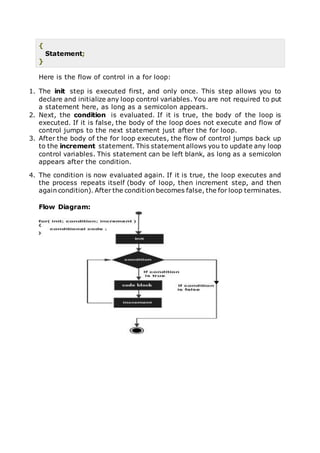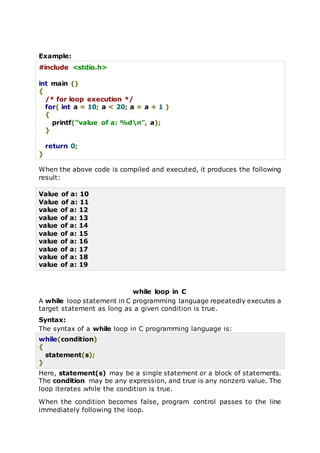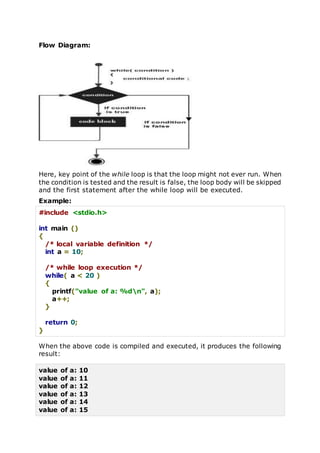Anúncio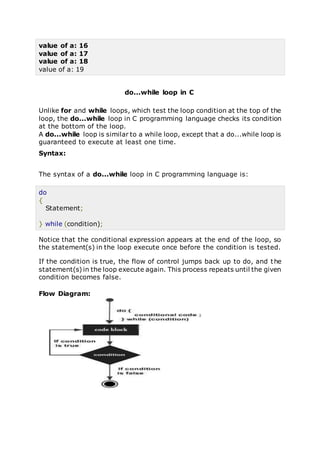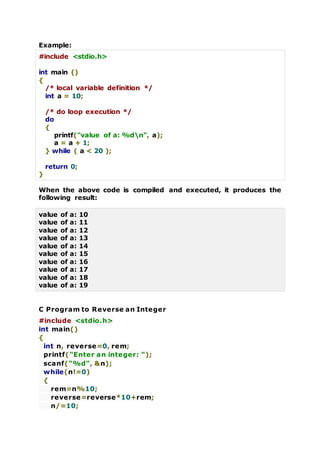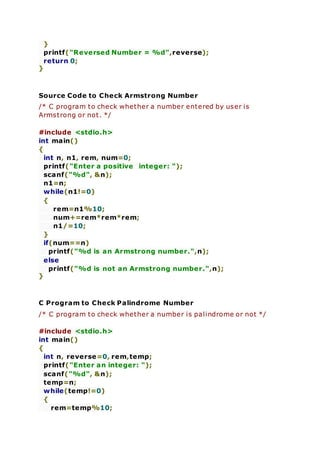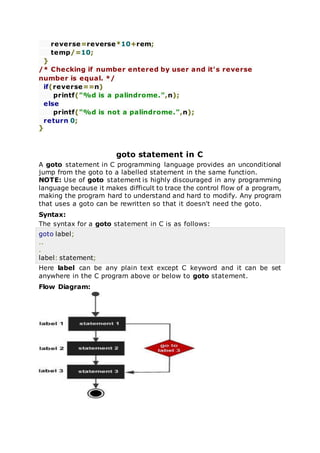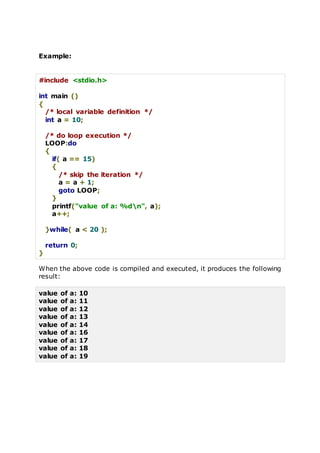Anúncio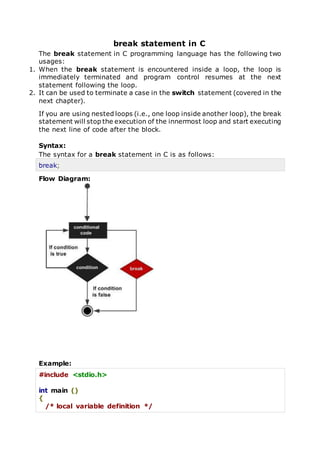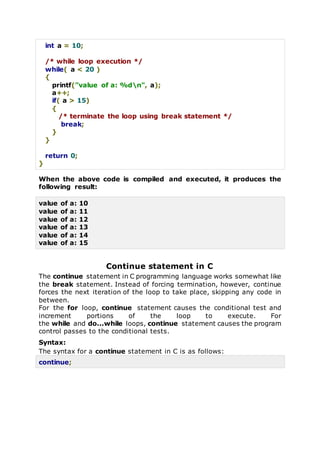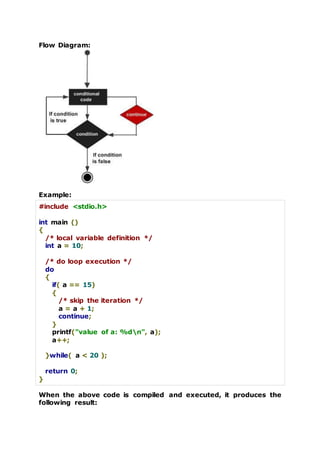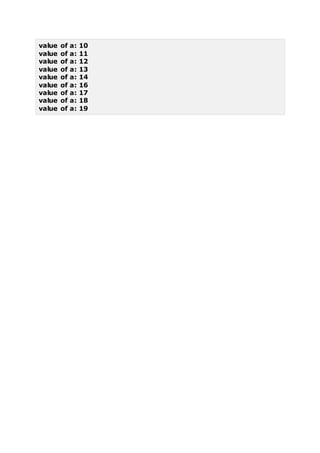Próximos SlideShares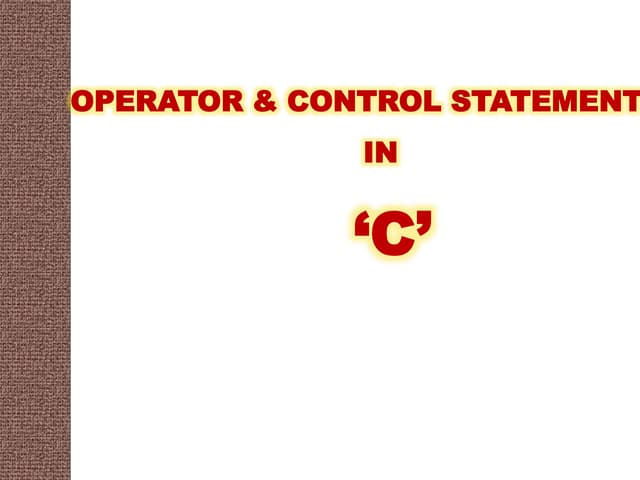Control statements in c
Carregando em ... 3
1 de 13
Anúncio

### Looping statements

1. C – Loop control statements Loop control statements in C are used to perform looping operations until the given condition is true. Control comes out of the loop statements once condition becomes false. Types of loop control statements in C: There are 3 types of loop control statements in C language. They are, 1. for 2. while 3. do-while  Syntax for each C loop control statements are given in below table with description. S.no Loop Name Syntax Description 1 for for (exp1; exp2; expr3) { statements; } Where, exp1 – variable initialization ( Example: i=0, j=2, k=3 ) exp2 – condition checking ( Example: i>5, j<3, k=3 ) exp3 – increment/decrement ( Example: ++i, j–, ++k ) 2 while while (condition) { statements; } where, condition might be a>5, i<10 3 do while do { statements; } while (condition); where, condition might be a>5, i<10 A for loop is a repetition control structure that allows you to efficiently write a loop that needs to execute a specific number of times. Syntax: The syntax of a for loop in C programming language is: for ( init; condition; increment )
2. { Statement; } Here is the flow of control in a for loop: 1. The init step is executed first, and only once. This step allows you to declare and initialize any loop control variables. You are not required to put a statement here, as long as a semicolon appears. 2. Next, the condition is evaluated. If it is true, the body of the loop is executed. If it is false, the body of the loop does not execute and flow of control jumps to the next statement just after the for loop. 3. After the body of the for loop executes, the flow of control jumps back up to the increment statement. This statement allows you to update any loop control variables. This statement can be left blank, as long as a semicolon appears after the condition. 4. The condition is now evaluated again. If it is true, the loop executes and the process repeats itself (body of loop, then increment step, and then again condition). After the condition becomes false, the for loop terminates. Flow Diagram:
3. Example: #include <stdio.h> int main () { /* for loop execution */ for( int a = 10; a < 20; a = a + 1 ) { printf("value of a: %dn", a); } return 0; } When the above code is compiled and executed, it produces the following result: Value of a: 10 Value of a: 11 value of a: 12 value of a: 13 value of a: 14 value of a: 15 value of a: 16 value of a: 17 value of a: 18 value of a: 19 while loop in C A while loop statement in C programming language repeatedly executes a target statement as long as a given condition is true. Syntax: The syntax of a while loop in C programming language is: while(condition) { statement(s); } Here, statement(s) may be a single statement or a block of statements. The condition may be any expression, and true is any nonzero value. The loop iterates while the condition is true. When the condition becomes false, program control passes to the line immediately following the loop.
4. Flow Diagram: Here, key point of the while loop is that the loop might not ever run. When the condition is tested and the result is false, the loop body will be skipped and the first statement after the while loop will be executed. Example: #include <stdio.h> int main () { /* local variable definition */ int a = 10; /* while loop execution */ while( a < 20 ) { printf("value of a: %dn", a); a++; } return 0; } When the above code is compiled and executed, it produces the following result: value of a: 10 value of a: 11 value of a: 12 value of a: 13 value of a: 14 value of a: 15
5. value of a: 16 value of a: 17 value of a: 18 value of a: 19 do...while loop in C Unlike for and while loops, which test the loop condition at the top of the loop, the do...while loop in C programming language checks its condition at the bottom of the loop. A do...while loop is similar to a while loop, except that a do...while loop is guaranteed to execute at least one time. Syntax: The syntax of a do...while loop in C programming language is: do { Statement; } while (condition); Notice that the conditional expression appears at the end of the loop, so the statement(s) in the loop execute once before the condition is tested. If the condition is true, the flow of control jumps back up to do, and the statement(s) in the loop execute again. This process repeats until the given condition becomes false. Flow Diagram:
6. Example: #include <stdio.h> int main () { /* local variable definition */ int a = 10; /* do loop execution */ do { printf("value of a: %dn", a); a = a + 1; } while ( a < 20 ); return 0; } When the above code is compiled and executed, it produces the following result: value of a: 10 value of a: 11 value of a: 12 value of a: 13 value of a: 14 value of a: 15 value of a: 16 value of a: 17 value of a: 18 value of a: 19 C Program to Reverse an Integer #include <stdio.h> int main() { int n, reverse=0, rem; printf("Enter an integer: "); scanf("%d", &n); while(n!=0) { rem=n%10; reverse=reverse*10+rem; n/=10;
7. } printf("Reversed Number = %d",reverse); return 0; } Source Code to Check Armstrong Number /* C program to check whether a number entered by user is Armstrong or not. */ #include <stdio.h> int main() { int n, n1, rem, num=0; printf("Enter a positive integer: "); scanf("%d", &n); n1=n; while(n1!=0) { rem=n1%10; num+=rem*rem*rem; n1/=10; } if(num==n) printf("%d is an Armstrong number.",n); else printf("%d is not an Armstrong number.",n); } C Program to Check Palindrome Number /* C program to check whether a number is palindrome or not */ #include <stdio.h> int main() { int n, reverse=0, rem,temp; printf("Enter an integer: "); scanf("%d", &n); temp=n; while(temp!=0) { rem=temp%10;
8. reverse=reverse*10+rem; temp/=10; } /* Checking if number entered by user and it's reverse number is equal. */ if(reverse==n) printf("%d is a palindrome.",n); else printf("%d is not a palindrome.",n); return 0; } goto statement in C A goto statement in C programming language provides an unconditional jump from the goto to a labelled statement in the same function. NOTE: Use of goto statement is highly discouraged in any programming language because it makes difficult to trace the control flow of a program, making the program hard to understand and hard to modify. Any program that uses a goto can be rewritten so that it doesn't need the goto. Syntax: The syntax for a goto statement in C is as follows: goto label; .. . label: statement; Here label can be any plain text except C keyword and it can be set anywhere in the C program above or below to goto statement. Flow Diagram:
9. Example: #include <stdio.h> int main () { /* local variable definition */ int a = 10; /* do loop execution */ LOOP:do { if( a == 15) { /* skip the iteration */ a = a + 1; goto LOOP; } printf("value of a: %dn", a); a++; }while( a < 20 ); return 0; } When the above code is compiled and executed, it produces the following result: value of a: 10 value of a: 11 value of a: 12 value of a: 13 value of a: 14 value of a: 16 value of a: 17 value of a: 18 value of a: 19
10. break statement in C The break statement in C programming language has the following two usages: 1. When the break statement is encountered inside a loop, the loop is immediately terminated and program control resumes at the next statement following the loop. 2. It can be used to terminate a case in the switch statement (covered in the next chapter). If you are using nested loops (i.e., one loop inside another loop), the break statement will stop the execution of the innermost loop and start executing the next line of code after the block. Syntax: The syntax for a break statement in C is as follows: break; Flow Diagram: Example: #include <stdio.h> int main () { /* local variable definition */
11. int a = 10; /* while loop execution */ while( a < 20 ) { printf("value of a: %dn", a); a++; if( a > 15) { /* terminate the loop using break statement */ break; } } return 0; } When the above code is compiled and executed, it produces the following result: value of a: 10 value of a: 11 value of a: 12 value of a: 13 value of a: 14 value of a: 15 Continue statement in C The continue statement in C programming language works somewhat like the break statement. Instead of forcing termination, however, continue forces the next iteration of the loop to take place, skipping any code in between. For the for loop, continue statement causes the conditional test and increment portions of the loop to execute. For the while and do...while loops, continue statement causes the program control passes to the conditional tests. Syntax: The syntax for a continue statement in C is as follows: continue;
12. Flow Diagram: Example: #include <stdio.h> int main () { /* local variable definition */ int a = 10; /* do loop execution */ do { if( a == 15) { /* skip the iteration */ a = a + 1; continue; } printf("value of a: %dn", a); a++; }while( a < 20 ); return 0; } When the above code is compiled and executed, it produces the following result:
13. value of a: 10 value of a: 11 value of a: 12 value of a: 13 value of a: 14 value of a: 16 value of a: 17 value of a: 18 value of a: 19
Anúncio